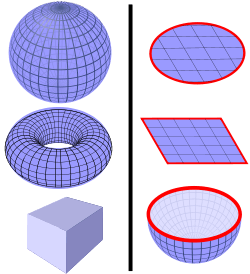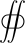# Gauss's law for magnetism

This article is about Gauss's law concerning the magnetic field. For analogous laws concerning different fields, see Gauss's law and Gauss's law for gravity. For Gauss's theorem, a mathematical theorem relevant to all of these laws, see Divergence theorem.

In physics, Gauss's law for magnetism is one of the four Maxwell's equations that underlie classical electrodynamics. It states that the magnetic field B has divergence equal to zero, in other words, that it is a solenoidal vector field. It is equivalent to the statement that magnetic monopoles do not exist. Rather than "magnetic charges", the basic entity for magnetism is the magnetic dipole. (Of course, if monopoles were ever found, the law would have to be modified, as elaborated below.)

Gauss's law for magnetism can be written in two forms, a differential form and an integral form. These forms are equivalent due to the divergence theorem.

The name "Gauss's law for magnetism" is not universally used. The law is also called "Absence of free magnetic poles". (or some variant); one reference even explicitly says the law has "no name". It is also referred to as the "transversality requirement" because for plane waves it requires that the polarization be transverse to the direction of propagation.

## Differential form

The differential form for Gauss's law for magnetism is:where ∇ · denotes divergence, and B is the magnetic field.

## Integral formDefinition of a closed surface.
Left: Some examples of closed surfaces include the surface of a sphere, surface of a torus, and surface of a cube. The magnetic flux through any of these surfaces is zero.
Right: Some examples of non-closed surfaces include the disk surface, square surface, or hemisphere surface. They all have boundaries (red lines) and they do not fully enclose a 3D volume. The magnetic flux through these surfaces is not necessarily zero.

The integral form of Gauss's law for magnetism states:where S is any closed surface (see image right), and dA is a vector, whose magnitude is the area of an infinitesimal piece of the surface S, and whose direction is the outward-pointing surface normal (see surface integral for more details).

The left-hand side of this equation is called the net flux of the magnetic field out of the surface, and Gauss's law for magnetism states that it is always zero.

The integral and differential forms of Gauss's law for magnetism are mathematically equivalent, due to the divergence theorem. That said, one or the other might be more convenient to use in a particular computation.

The law in this form states that for each volume element in space, there are exactly the same number of "magnetic field lines" entering and exiting the volume. No total "magnetic charge" can build up in any point in space. For example, the south pole of the magnet is exactly as strong as the north pole, and free-floating south poles without accompanying north poles (magnetic monopoles) are not allowed. In contrast, this is not true for other fields such as electric fields or gravitational fields, where total electric charge or mass can build up in a volume of space.

## Vector potential

Due to the Helmholtz decomposition theorem, Gauss's law for magnetism is equivalent to the following statement:

There exists a vector field A such that.

The vector field A is called the magnetic vector potential.

Note that there is more than one possible A which satisfies this equation for a given B field. In fact, there are infinitely many: any field of the form φ can be added onto A to get an alternative choice for A, by the identity (see Vector calculus identities):since the curl of a gradient is the zero vector field:This arbitrariness in A is called gauge freedom.

## Field lines

Main article: Field line

The magnetic field B, like any vector field, can be depicted via field lines (also called flux lines) – that is, a set of curves whose direction corresponds to the direction of B, and whose areal density is proportional to the magnitude of B. Gauss's law for magnetism is equivalent to the statement that the field lines have neither a beginning nor an end: Each one either forms a closed loop, winds around forever without ever quite joining back up to itself exactly, or extends to infinity.

## Modification if magnetic monopoles exist

Main article: Magnetic monopole

If magnetic monopoles were discovered, then Gauss's law for magnetism would state the divergence of B would be proportional to the magnetic charge density ρm, analogous to Gauss's law for electric field. For zero net magnetic charge density (ρm = 0), the original form of Gauss's magnetism law is the result.

The modified formula in SI units is not standard; in one variation, magnetic charge has units of webers, in another it has units of ampere-meters.

Units Equation
cgs unitsSI units (weber convention)SI units (ampere-meter convention)where μ0 is the vacuum permeability.

So far no magnetic monopoles have been found, despite extensive search.

## History

This idea, of the nonexistence of magnetic monopoles, originated in 1269 by Petrus Peregrinus de Maricourt. His work heavily influenced William Gilbert, whose 1600 work De Magnete spread the idea further. In the early 1800s Michael Faraday reintroduced this law, and it subsequently made it into James Clerk Maxwell's electromagnetic field equations.

7. Moulin, F. (2001). "Magnetic monopoles and Lorentz force". Il Nuovo Cimento B. 116 (8): 869–877. arXiv:math-ph/0203043. Bibcode:2001NCimB.116..869M.
9. See for example equation 4 in Nowakowski, M.; Kelkar, N. G. (2005). "Faraday's law in the presence of magnetic monopoles". Europhysics Letters. 71 (3): 346. arXiv:physics/0508099. Bibcode:2005EL.....71..346N. doi:10.1209/epl/i2004-10545-2.